# Calculate the following, given A âRR3 + ае4 sin and B-bR3 + b 2 12 sin...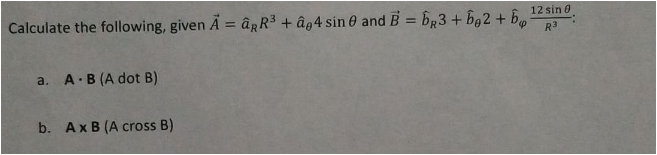Calculate the following, given A âRR3 + ае4 sin and B-bR3 + b 2 12 sin 0 a. A B (A dot B) b, A x B (A cross B)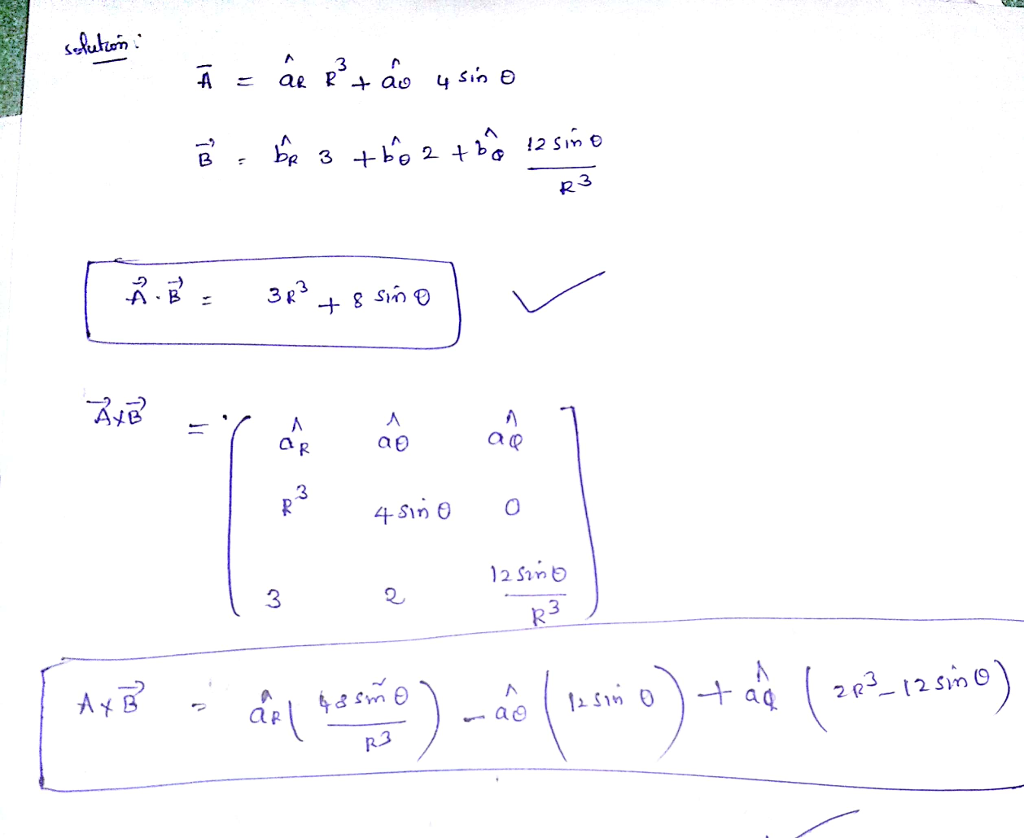#### Earn Coin

Coins can be redeemed for fabulous gifts.

Similar Homework Help Questions
• ### The only ideas that can be used include: area ABCI-RA2(A+B+C-lpi), the Pythagorean theorem: Cos c cos a cos b. Vectors-dot product cross product, sin A-sin a/sin c; cos A-cos a sin b/sin c; spher...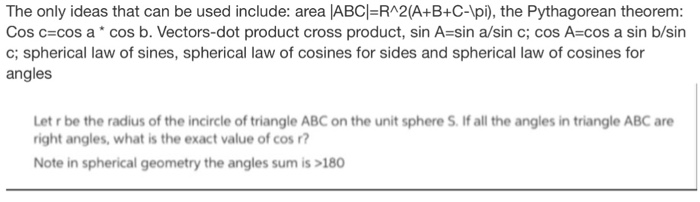The only ideas that can be used include: area ABCI-RA2(A+B+C-lpi), the Pythagorean theorem: Cos c cos a cos b. Vectors-dot product cross product, sin A-sin a/sin c; cos A-cos a sin b/sin c; spherical law of sines, spherical law of cosines for sides and spherical law of cosines for angles Let r be the radius of the incircle of triangle ABC on the unit sphere S. If all the angles in triangle ABC are right angles, what is the exact...

• ### a) Find sin( 2 ) + cos(20) using the picture below. 0 12 b) Find the...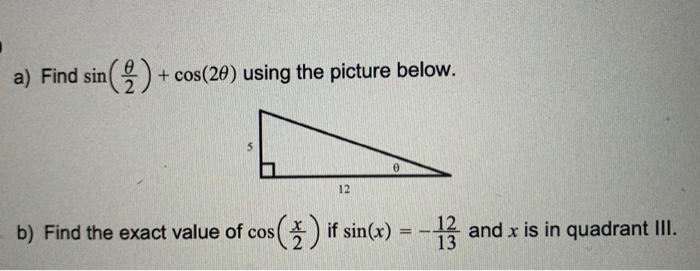a) Find sin( 2 ) + cos(20) using the picture below. 0 12 b) Find the exact value of cos s(3) if sin(x) = 13 and x is in quadrant III.

• ### SIII 4. Evaluate 3 (cos 30°)2 +2 (sin 60°)2 without using a calculator. 12. Given that...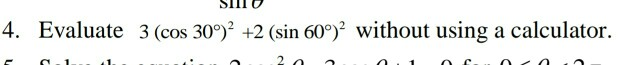SIII 4. Evaluate 3 (cos 30°)2 +2 (sin 60°)2 without using a calculator. 12. Given that sin 0 =-. Show that sin (90° — O)=cos .

• ### 10. Rewrite the following expressions with exponents no greater than 1: A. sin? 2 B. cost...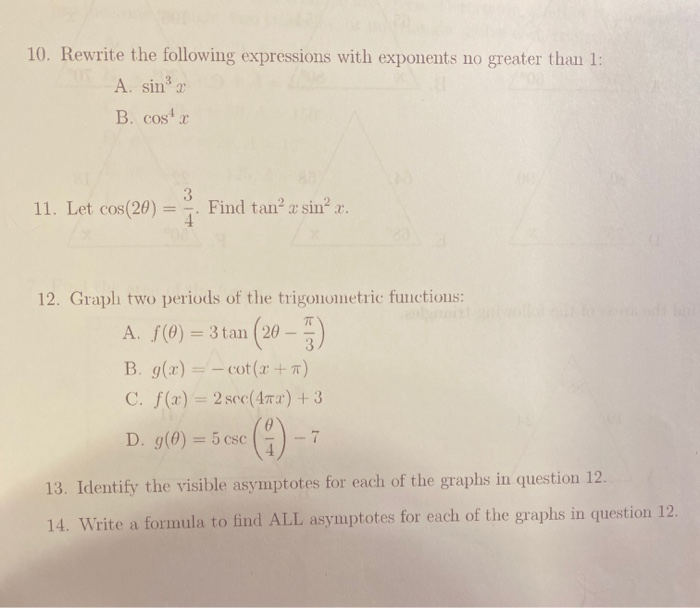10. Rewrite the following expressions with exponents no greater than 1: A. sin? 2 B. cost 11. Let cos(20) 3 4 Find tan’ a sin 12. Graph two periods of the trigonometric functions: A. f(0) = 3 tan (20 – 5). B. g(x) = – cot(.x+7) C. f(x) = 2 sec(47x) + 3 D. g(0) = 5 csc -7 13. Identify the visible asymptotes for each of the graphs in question 12. 14. Write a formula to find ALL asymptotes...

• ### Find the exact value of sin(a - b) if sin a = 15 12 17 and...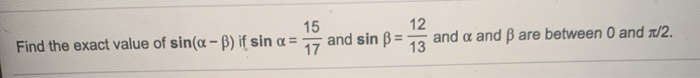Find the exact value of sin(a - b) if sin a = 15 12 17 and sin B = and a and Bare between 0 and 1/2. 13

• ### Calculate the area between the graphs of y = sin(2 · x) and y = 2...

Calculate the area between the graphs of y = sin(2 · x) and y = 2 · sin(x) on [0, π].

• ### II- Given 2 brass specimens with initial dimensions as follo Specimen #1: rectangular cross-section (12 x...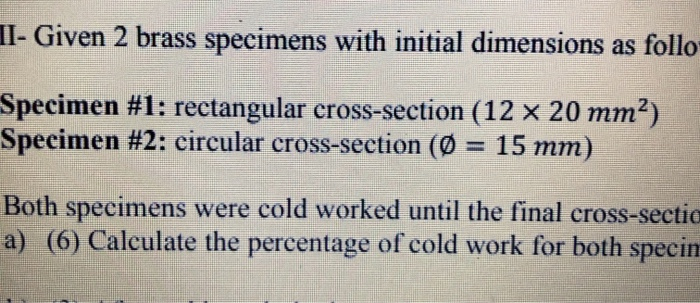II- Given 2 brass specimens with initial dimensions as follo Specimen #1: rectangular cross-section (12 x 20 mm) Specimen #2: circular cross-section (0 = 15 mm) Both specimens were cold worked until the final cross-sectia a) (6) Calculate the percentage of cold work for both specin

• ### Go Help we ar given a funchon f (x) = sin 2x 2 ing a Calculate he cosive sevies IT ntervs Sketch the even extend ,f -IT, TT) Go Help we ar given a funchon f (x) = sin 2x 2 ing a Calculate he...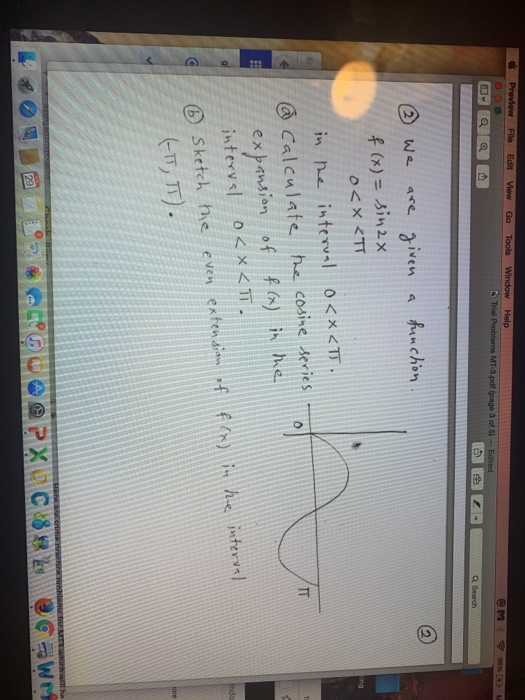Go Help we ar given a funchon f (x) = sin 2x 2 ing a Calculate he cosive sevies IT ntervs Sketch the even extend ,f -IT, TT) Go Help we ar given a funchon f (x) = sin 2x 2 ing a Calculate he cosive sevies IT ntervs Sketch the even extend ,f -IT, TT)

• ### Given an angle 0° s < 360°, a. If I calculate sin (), am I calculating...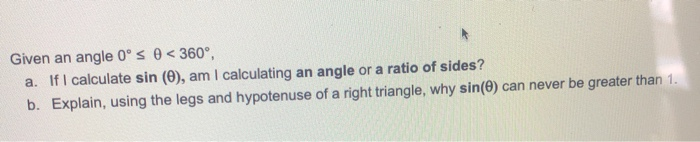Given an angle 0° s < 360°, a. If I calculate sin (), am I calculating an angle or a ratio of sides? b. Explain, using the legs and hypotenuse of a right triangle, why sin(e) can never be greater than 1.

• ### 12 8 Calculate sin V2 2 N Show transcribed image text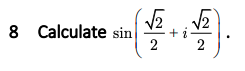12 8 Calculate sin V2 2 N

Free Homework App